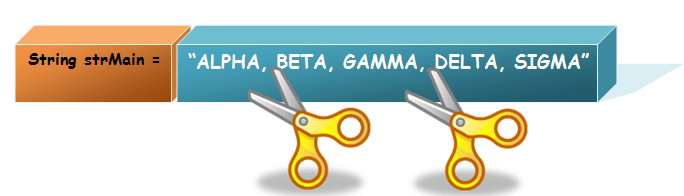Go to start of banner

# Java Split String

While programming, we may need to break a string based on some attributes. Mostly this attribute will be a separator or a common - with which you want to break or split the string.

The `StrSplit()` method splits a String into an array of substrings given a specific delimiter.

## Syntax

```public String split(String regex)
public String split(String regex, int limit)  ```

Parameter

• Regex: The regular expression is applied to the text/string
• Limit: A limit is a maximum number of values the array. If it is omitted or zero, it will return all the strings matching a regex.

## Split String Example

Suppose we have a string variable named `strMain` formed of a few words like Alpha, Beta, Gamma, Delta, Sigma – all separated by the comma (,).Here if we want all individual strings, the best possible pattern would be to split it based on the comma. So we will get five separate strings as follows:

• Alpha
• Beta
• Gamma
• Delta
• Sigma

Use the split method against the string that needs to be divided and provide the separator as an argument.

In this case, the separator is a comma (,) and the result of the split operation will give you an array split.

```class StrSplit{
public static void main(String []args){
String strMain = "Alpha, Beta, Delta, Gamma, Sigma";
String[] arrSplit = strMain.split(", ");
for (int i=0; i < arrSplit.length; i++)
{
System.out.println(arrSplit[i]);
}
}
}```

The loop in the code just prints each string (element of array) after the split operation, as shown below-

Output:

Output

Alpha

Beta

Delta

Gamma

Sigma

## Example: Java String split() method with regex and length

Consider a situation, wherein you require only the first ‘n’ elements after the split operation but want the rest of the string to remain as it is. An output something like this-

1. Alpha
2. Beta
3. Delta, Gamma, Sigma

This can be achieved by passing another argument along with the split operation, and that will be the limit of strings required.

Consider the following code –

```class StrSplit2{
public static void main(String []args){
String strMain = "Alpha, Beta, Delta, Gamma, Sigma";
String[] arrSplit_2 = strMain.split(", ", 3);
for (int i=0; i < arrSplit_2.length; i++){
System.out.println(arrSplit_2[i]);
}
}
}```

Output:

Output

Alpha

Beta

Delta, Gamma, Sigma

## How to Split a String by Space

Consider a situation, wherein you want to split a string by space. Let’s consider an example here; we have a string variable named strMain formed of a few words Welcome to Java.

```public class StrSplit3{
public static void main(String args[]){
String strMain ="Welcome to Java";
String[] arrSplit_3 = strMain.split("\\s");
for (int i=0; i < arrSplit_3.length; i++){
System.out.println(arrSplit_3[i]);
}
}
}```

Output:

Output

Welcome

to

Java

• No labels
Write a comment…